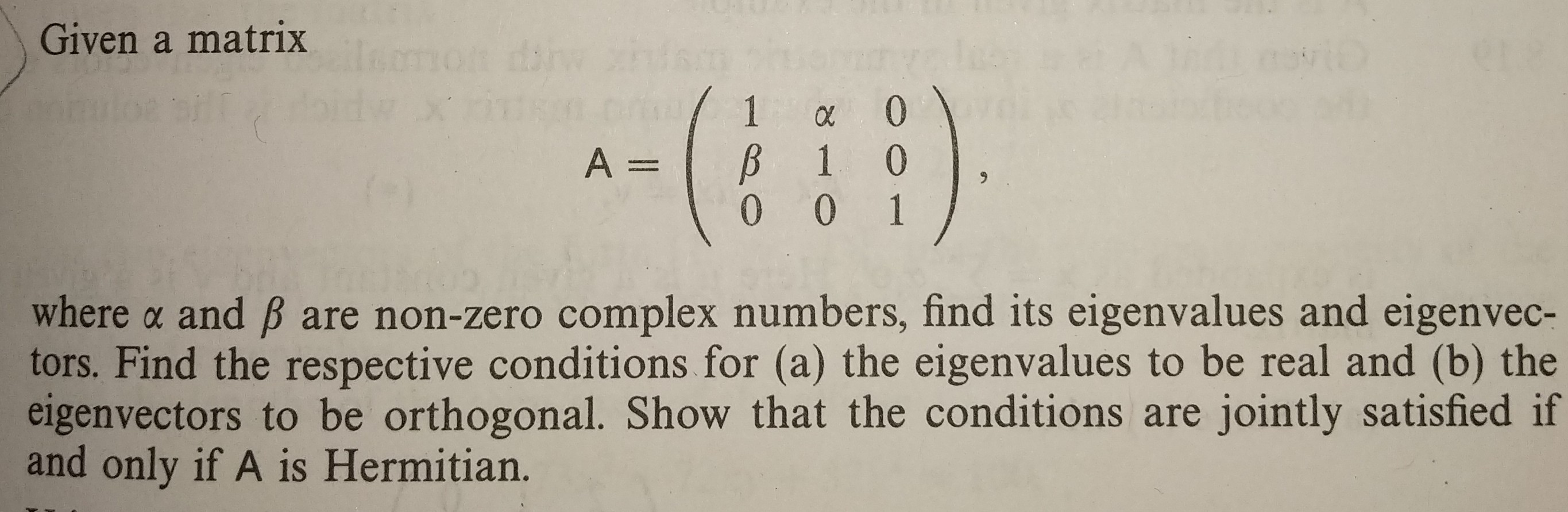# Given a matrix where α and β are non-zero complex numbers, find its eigenvalues and eigenvec- tors. Find the respective conditions for (a) the eigenvalues to be real and (b) the eigenvectors to be orthogonal. Show that the conditions are jointly satisfied if and only if A is Hermitian.

Question
501 viewshelp_outlineImage TranscriptioncloseGiven a matrix where α and β are non-zero complex numbers, find its eigenvalues and eigenvec- tors. Find the respective conditions for (a) the eigenvalues to be real and (b) the eigenvectors to be orthogonal. Show that the conditions are jointly satisfied if and only if A is Hermitian. fullscreen

### Want to see the step-by-step answer?